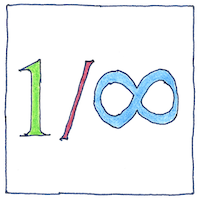# 1656 Infinite and infinitesimal

## The book of science

Tom Sharp

 John Wallis mathematics## Infinite and infinitesimal

• In 1635, Bonaventura Cavalieri
• introduced infinitesimals,
• which he called indivisibles,
• as a means of calculating areas and volumes.
• John Wallis’s treatise on conic sections
• clarified Decartes’ work on analytic geometry
• and introduced the symbol ∞ for infinity
• and the symbol 1/∞ for the infinitesimal.
• Wallis’s work Arithmetica Infinitorum
• finds the area under a curve by integration
• and established the principle of interpolation
• to derive the value of π.
• Thus Wallis, James Gregory, and Isaac Barrow
• contributed to the development of integral calculus
• put to use by Isaac Newton and given
• modern notation by Gottfried Wilhelm Leibniz.

## Infinity symbol

• The infinity symbol, ∞,
• the digit 8 on its side,
• traces a closed path
• without an end.
• More could be said
• approaching the symbol
• but not what it symbolizes
• unless turning into itself.

## Negative numbers

• John Wallis introduced the number line
• and interpreted negative numbers
• as values greater than infinity
• rather than as less than nothing.
• Me, I think of negative numbers
• as mere arithmetic conveniences
• and I’ve learned there are
• positive infinities greater than infinity.

James Gregory stated and proved the fundamental theorem of calculus for a special class of curves; Isaac Barrow proved the fundamental theorem in general, showing that differentiation and integration are inverses of each other. Isaac Newton was a student of Isaac Barrow.

See also in The book of science: